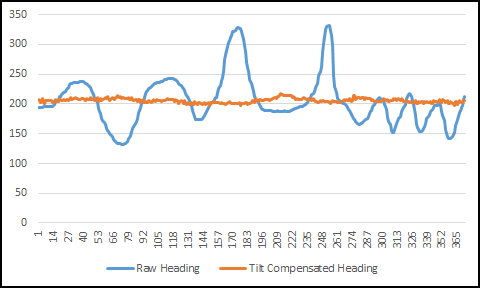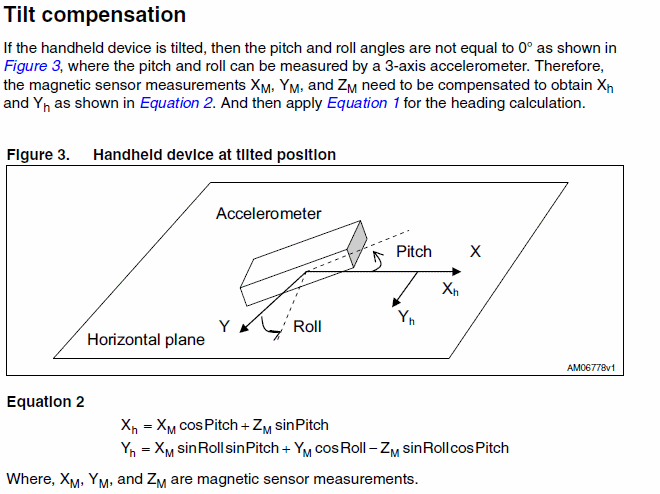# Create a Digital Compass with the Raspberry Pi - Part 2 - "Tilt Compensation"

This part 2 of a multi part series on how to use a digital compass(magnetometer) with your Raspberry Pi.

Part 1 can be found here and is a prerequisite to part 2.

# Code for this guide

Git repository here
The code can be pulled down to your Raspberry Pi with;

pi@raspberrypi ~ \$ git clone https://github.com/mwilliams03/BerryIMU.git

The code for this guide can be found under the compass_tutorial02_tilt_compensation directory.

# Calculating Compass Heading

Part 1 covered how to get the heading from the magnetometer, however this is only reliable when the magnetometer is on a flat surface.  If the magnetometer is tilted, the heading will skew and not be correct.

The chart below shows a compass being held at 200 degrees and being tilted in various directions. The blue line is the raw heading, the orange line is the heading after applying tilt compensation. As you can see,  without tilt compensation the heading will change if the compass is tilted.The below image is from a page from application note  AN3192 from ST, the manufacture of the LSM9DS0 which is the magnetometer and accelerometer we are using in this guide. This application note goes into detail on how to calculate a tilt compensated heading.In summary, it states that if the magnetometer is at a level position, then the only calculation that is needed to be performed is;

`heading = 180*atan2(magRawY,magRawX)/M_PI;`

If the magnetometer is tilted, we need to factor in the tilt values to get an accurate heading. This is what the application note says about this;In summary; We will use an accelerometer to calculate pitch & roll which will then be included in our formula to calculate the heading with tilt compensation. Here is the formula;

```float magXcomp = mag_raw*cos(asin(accXnorm))+mag_raw*sin(pitch);
float magYcomp = mag_raw[o]*sin(asin(accYnorm/cos(pitch)))*sin(asin(accXnorm))+mag_raw*cos(asin(accYnorm/cos(pitch)))-mag_raw*sin(asin(accYnorm/cos(pitch)))*cos(asin(accXnorm));

The above formula looks very complex, you don't really need to understand it and we do simplify it further on in this post.
One point to note ;The arcsin function has good linearity between about -45º to +45º, so the accuracy of the
pitch and roll calculation degrades when tilt angles exceed this range# Reading Values from the Accelerometer

Most accelerometers are on the same chip as a magnetometer, which is in this instance a LSM9DS0 on a BerryIMU.  In some instances the i2c address of the accelerometer will be different than the magnetometer (E.g on the LSM303DLHC).  On the LSM9DS0 , the i2c address is the same for both accelerometer and magnetometer.

For the BerryIMU, the default address is 0x1E.

We will create a new function to write to the accelerometer;

```void writeMagReg(uint8_t reg, uint8_t value)
{
int result = i2c_smbus_write_byte_data(file, reg, value);
if (result == -1)
{
printf ("Failed to write byte to I2C Mag.");
exit(1);
}
}

```

And we will also create a new function to read from the accelerometer. Reading values from the accelerator is similar to reading values from the magnetometer, we will read a block of 6 bytes.

```void readACC(int  *a)
{
uint8_t block;

readBlock(0x80 | OUT_X_L_A, sizeof(block), block);

*a = (int16_t)(block | block << 8);
*(a+1) = (int16_t)(block | block << 8);
*(a+2) = (int16_t)(block | block << 8);
}
```

Here is what is happening in the readACC() function;
1) An array of 6 bytes is first created to store the values.
2) Using the readBlock() function (created in part 1), we read 6 bytes starting at OUT_X_L_A (0x28). This is shown on page 61 of the datasheet.
3) The values are expressed in 2’s complement (MSB for the sign and then 15 bits for the value) so we need to combine;
block & block for X axis
block & block for Y axis
block & block for Z axis

Working with the code we created in part 1, we now need to add code to enable the accelerometer;

``` // Enable accelerometer.
writeAccReg(CTRL_REG1_XM, 0b01100111);
writeAccReg(CTRL_REG2_XM, 0b00100000);
```

CTRL_REG1_XM tells the accelerometer to enable all axis, set it to continuous update mode and a data rate of 100Hz - Page 55 of the Datasheet.
CTRL_REG2_XM,  will set the accelerometer to +/-16 gauss full scale.

# The Math

First, we need to normalize the raw accelerometer data;

```accXnorm = accRaw/sqrt(accRaw* accRaw+ accRaw * accRaw + accRaw * accRaw);
accYnorm =accRaw/sqrt(accRaw *accRaw + accRaw * accRaw + accRaw * accRaw);
```

As the pitch and roll is calculated a number of times in the tilt compensation formula, we will split these off and perform both calculations once beforehand;

```	pitch = asin(accXnorm);
roll = -asin(accYnorm/cos(pitch));
```

We now calculate the new tilt compensated magnetometer readings;

```magXcomp = *mag_raw*cos(pitch)+*(mag_raw+2)*sin(pitch);
magYcomp = *mag_raw*sin(roll)*sin(pitch)+*(mag_raw+1)*cos(roll)-*(mag_raw+2)*sin(roll)*cos(pitch);
```

We then use these new compensated values in our heading formula;

```	heading = 180*atan2(magYcomp,magXcomp)/M_PI;
```

We will also convert the values so that they are in the range of 0 to 360;

```if(heading < 0)

```

# Display the New Heading

After the last calculation, we can add another print statement to print out the new compensated heading.

```                printf("Compensated  Heading %7.3f  \n", heading);
```

When you now run the program, you will notice that the heading stays the same value if the magnetometer is tilted to within 40 degrees.

RAW heading in Degrees 172.000 ##### Tilt Comp heading 170.043
RAW heading in Degrees 170.044 ##### Tilt Comp heading 168.015
RAW heading in Degrees 167.341 ##### Tilt Comp heading 164.817
RAW heading in Degrees 163.133 ##### Tilt Comp heading 172.429
RAW heading in Degrees 155.924 ##### Tilt Comp heading 162.065
RAW heading in Degrees 149.081 ##### Tilt Comp heading 153.045
RAW heading in Degrees 144.548 ##### Tilt Comp heading 152.323
RAW heading in Degrees 139.844 ##### Tilt Comp heading 157.862
RAW heading in Degrees 135.603 ##### Tilt Comp heading 171.924
RAW heading in Degrees 130.305 ##### Tilt Comp heading 172.325
RAW heading in Degrees 122.636 ##### Tilt Comp heading 146.452
RAW heading in Degrees 118.951 ##### Tilt Comp heading 143.692

# Compiling

pi@raspberrypi ~ \$ gcc compass_tutorial01.c -o compass_tutorial01 -lm

# Upside down IMU

If your IMU is upside down, you will need to invert X & Y accelerator values for the tilt compensation to work.
Just add the two lines below right after the raw values have been read from the accelerometer.

```              accRaw = -accRaw;
accRaw = -accRaw;

```## 14 thoughts on “Create a Digital Compass with the Raspberry Pi - Part 2 - "Tilt Compensation"”

1. Hello and thank you for developing this IMU and code! I plan to use the IMU on a GPS guided vehicle.

While running the code from this tutorial, I think I found a typo. The compensation in roll was not working properly. I don't pretend to understand these equations but I noticed that the roll calculation is negated when it looks like it should not be. In the code snippet shown near the top of this page, before you break off the separate calculations of pitch and roll, the calculation for magYcomp looks like this:

magYcomp = mag_raw * sin(asin(accYnorm/cos(pitch))) * sin(asin(accXnorm)) + mag_raw * cos(asin(accYnorm/cos(pitch))) - mag_raw * sin(asin(accYnorm/cos(pitch))) * cos(asin(accXnorm));

So, every where roll is needed above (3 times) you have:

asin(accYnorm / cos(pitch)

But on line 103 from the git repo where you pre-compute roll, you have this:

roll = -asin(accYnorm / cos(pitch));

So, taking out this negation, I get good results in both pitch and roll now.

Hope this helps!

1. I should make the code clearer;
When the IMU is facing the correct way up, this is when the chips would be on the tops side of the PCB, use this;
`roll = -asin(accYnorm / cos(pitch));`

When the IMU is upside down, this is when it is sitting directly on top of the GPIO headers and the chips are on the underside of the PCB. Use this
`roll = asin(accYnorm / cos(pitch));`

Maybe you can test to confirm?

1. OK. that makes sense then. Actually, what I found was when the chip is down, as when mounted to an RPi, I use the negated roll calculation for it to work and positive otherwise. Thanks!

2. Thanks for your tutorial! It is really great. Suggestion for a next tutorial: measure distance travelled so I can follow my cat :). Or does anyone have any info on how to do that?

3. Robert says:

Hi,
I am currently on step 2 and am experiencing an error after compiling and running compass_tutorial02. When I run the program, all of the heading and compensated heading values are the same for all time values; all I get when I run the program is two columns with the same two heading numbers repeating over and over. I am a novice when it comes to coding, do you have any thoughts on what might be wrong?
Thank you very much for your help.

1. Robert says:

Please disregard the above question. I had wrong device addresses. Thanks

4. Will says:

What is your reference for the math calculations? Most other examples/tutorials/refs I see online are different.

1. From the ST application not.
show me one of those examples and I can tell you why

5. Andrew says:

I am having some problems with the accuracy of the heading values from this code. It doesn't seem right since when I turn it one way the heading decreases to a certain point then it begins to increase again.

1. to get accurate readings, you need to calibrate the compass.
Our GIT repo has code for this.

6. rhys horner says:

This is a great tutorial thank you so very much for sharing this.
I have learned so much about the theory, operation and calculations involved in AHRS compass.

I have an issue with the heading while roll is applied.
the heading seems reasonable while the board is flat and level and while there is pitch applied, but when roll is applied the heading appears to be almost the same through all the different headings the heading measurement only changes through about 30°

7. Boris Koerber says:

Thank you very much for this extremely well made instruction, Mark!

Some time ago, I built an inclinometer using the ADXL345 module with an arduino. It had the feature to auto-level via pressing a button. So when putting it into my car, I don't have to care about aligning the accelerometer module. Just place it where I want it, press the auto-level button and it sets the actual position as pitch=0 and roll=0.

Is this possible with your setup as well and if yes, how? If you want, I could send you my arduino C-code for understanding my process.

Best regards,
Boris

8. Muhammad Jumshed says:

Hi ,
This code gives discontinuities very frequently in roll for LSM9DS1. Pitch works fine. Could you please advise me?
I used another formula from some other source
float roll = atan2(ay, sqrt(ax * ax + az * az));
and that makes the roll work fine.. Could you please put some light on it.
Thanks,

1. Jumshed Akhtar says:

Sorry, there was an error in my code while porting. so it is working now..
But I could not understand the why different people are deriving different formulas for pith and roll and tilt compensation. Thanks,

This site uses Akismet to reduce spam. Learn how your comment data is processed.